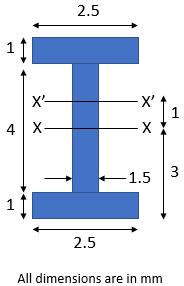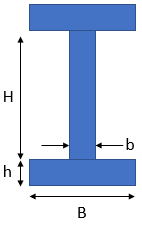## Parallel Axis

In the below figure, what is the area moment of inertia about the X'-X' axis, which is 1 mm higher from the centroid?Hint
The area moment of inertia of an I-Beam along the horizontal axis passing through the body's centroid:
$$I_{xx}=\frac{H^3b}{12}+2\left ( \frac{h^3B}{12}+\frac{hB(H+h)^2}{4} \right )$$$where $$H$$ is the flange-to-flange inner face height, $$B$$ is the flange's width, $$h$$ is the flange's thickness, and $$b$$ is the web thickness. Hint 2 Parallel Axis Theorem: $$I_x=I_{x_{c}}+d_{y}^{2}A$$$
where $$d_y$$ is the distance between the new axis and the object’s centroid, $$I_{x_{c}}$$ is the moment of inertia about the centroid axis, $$A$$ is the total cross sectional area, and $$I_x$$ is the moment of inertia about the new axis
The area moment of inertia of an I-Beam along the horizontal axis passing through the body's centroid:
$$I_{xx}=\frac{H^3b}{12}+2\left ( \frac{h^3B}{12}+\frac{hB(H+h)^2}{4} \right )$$$where $$H$$ is the flange-to-flange inner face height, $$B$$ is the flange's width, $$h$$ is the flange's thickness, and $$b$$ is the web thickness.$$I_{xx}=\frac{4^3(1.5)}{12}+2\left ( \frac{1^3(2.5)}{12}+\frac{1(2.5)(4+1)^2}{4} \right )$$$
$$=8+2\left ( 0.2083+15.625 \right )=39.67\approx 40\:mm^4$$$Because we want to find the moment of inertia about the parallel axis (X'-X') that is offset from the centroid (X-X) by 1 mm, we must use the Parallel Axis Theorem: $$I_x=I_{x_{c}}+d_{y}^{2}A$$$
where $$d_y$$ is the distance between the new axis and the object’s centroid, $$I_{x_{c}}$$ is the moment of inertia about the centroid axis, $$A$$ is the total cross sectional area, and $$I_x$$ is the moment of inertia about the new axis.
$$I_{x'x'}=40mm^4+(1mm)^{2}[(2.5)(1)+(4)(1.5)+(2.5)(1)]mm^2$$$$$I_{x'x'}=40mm^4+11mm^4=51\:mm^4$$$
$$51\:mm^4$$\$